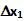## Sunday, July 4, 2010

### Irodov Problem 4.25Let l1 and l2 be the rest lengths of the two springs. When the mass is suspended, it will cause the springs to elongate and support the hanging mass. when the springs + mass system is in equilibrium, let the elongations of the two springs be x1 and x2. The total elongation of the two springs put together will be,Perhaps the most important concept to understand in solving this problem is to understand that the tension in both the springs must be exactly the same if the mass of the springs is zero. To understand why, let us assume that each of the springs have different tensions T1 and T2. Then, the net force acting on the section AB will be T1 - T2. Since the mass of this section is zero T1 must be equal to T2 since otherwise the section will undergo an infinite acceleration.

At equilibrium,There are two forces acing on the mass i) the tension in the spring T2 and ii) the force of gravity mg. Since the mass is at equilibrium,Now consider the state when the mass is pulled a distancefrom its equilibrium position. Let the respective elongations for the springs from their equilibrium positions beand. Then we have,Using the same argument as before,Now the equation of motion of the mass can be written as,